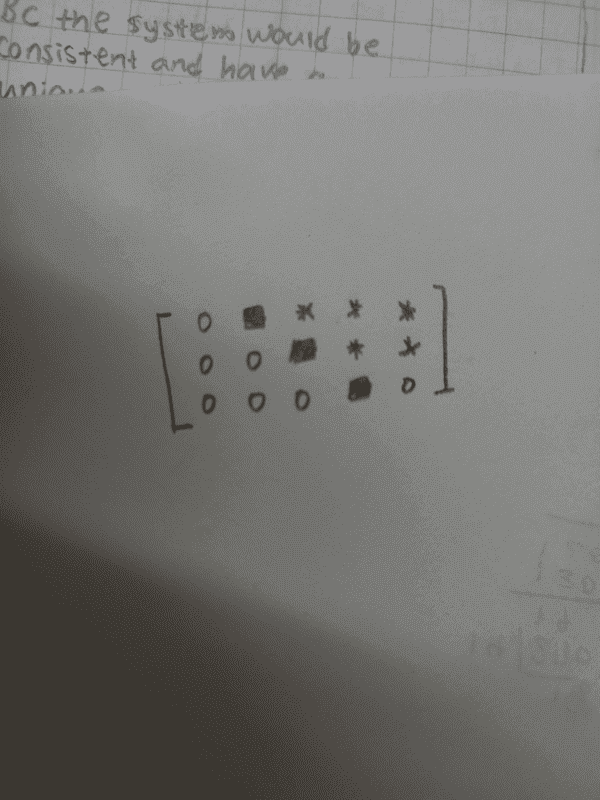# Linear Algebra uniqueness of solution

• Sunwoo Bae
In summary, the system described has a solution due to the lack of rows in the form of [0000b]. The first column consists of all 0s, making x1 a free variable. Since systems with free variables have infinite solutions, the solution is not unique. Therefore, the matrix is consistent and has no unique solutions. The notation used includes squares representing leading entries (which do not have to be the same number) and stars representing any numbers (which also do not have to be the same numbers). The matrix shown is an augmented matrix, and the lack of a zero row is not sufficient to determine the solution unless it is in row reduced echelon form.

#### Sunwoo Bae

Homework Statement
determine if the system is consistent. If the system is consistent, determine if the solution is unique
Relevant Equations
noneMy guess is that since there are no rows in a form of [0000b], the system is consistent (the system has a solution).
As the first column is all 0s, x1 would be a free variable.
Because the system with free variable have infinite solution, the solution is not unique.
In this way, the matrix is consistent, and has no unique solutions.

Would this be a correct answer, and is the explanation suitable?

Thank you!

Can you explain the notation a little bit more here? are all the *s intended to be the same number? Are all the little boxes intended to be the same number?

How do you get from a matrix to a system of equations? Is the matrix written as [A|b] for the system of equations Ax=b?

Office_Shredder said:
Can you explain the notation a little bit more here? are all the *s intended to be the same number? Are all the little boxes intended to be the same number?

How do you get from a matrix to a system of equations? Is the matrix written as [A|b] for the system of equations Ax=b?
The square represents leading entries (they do not have to be the same number). the star represents any numbers (the stars do not have to be the same numbers). 0 is the number 0.

The matrix above is the augmented matrix.

Sorry for the lack of explanation in the question...

I think you are entirely correct

Sunwoo Bae said:
The square represents leading entries (they do not have to be the same number). the star represents any numbers (the stars do not have to be the same numbers). 0 is the number 0.

The matrix above is the augmented matrix.

Sorry for the lack of explanation in the question...
Is there more to say about the entries? Are all the stars and squares non-zero? It seems like it would be easy to make the first two rows contradictory and therefore no solution.

I think the squares are intended to be non zero (that's the definition of a leading entry) but the stars could be zero.

I also agree with the answer, as long as the context is obvious that you are talking about a row reduced echelon form of a matrix (if not, you might want to say something about it, since the lack of a zero row is not sufficient)

•FactChecker
Office_Shredder said:
I think the squares are intended to be non zero (that's the definition of a leading entry)
Good point. I missed that. Thanks.